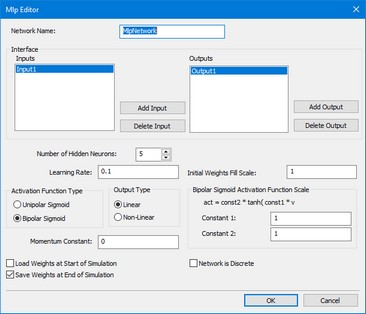﻿ 20-sim webhelp > Toolboxes > Control Toolbox > MLP Network Editor > How to use the MLP Editor

# How to use the MLP Editor

The MLP Editor can be used to define a 20-sim Multi Layer Perceptron (MLP) network. The editor is opened when you try to edit this submodel (using the Go Down command).## General Settings

 • Network Name: this is the local name of the submodel representing the MLP network (this option is not yet supported).
 • Number Hidden Neurons: The MLP network specified by this editor has one layer of hidden neurons. You can specify here the number of neurons that should be used.
 • Learning Rate: Specify the learning rate that should be used during training. If you do not want the network to learn, enter a zero value here.
 • Initial Weights Fill Scale: To start proper learning of the network, all initial weights will be given a random value (unequal to zero!) between -scale and +scale. You can enter the scale factor here.
 • Activation Function Type: Select one of the following activation functions
 • Unipolar Sigmoid: act = 1/(1 + exp(-v) )
 • Bipolar Sigmoid: act = const2*tanh(const1*v)
 • Bipolar Sigmoid Activation Function Scale: If a Bipolar Sigmoid was chosen as the activation function, you can enter here the values of const1 and const2.
 • Momentum Constant: Select the momentum constant here.
 • Load Weights at Start of Simulation: Select this option, if you want to use predefined weights stored on file (saved in a previous run). Before each simulation run, you will be asked to enter the filename of this weights file.
 • Save Weights at Start of Simulation: Select this option, if you want to store the weights on file. After each simulation run, you will be asked to enter the filename of this weights file.
 • Network is Discrete: Select this option if the B-spline network is connected with discrete-time models. Deselect this option if the network is connected with continuous models.

## Inputs

 • Add Input: Add a new input to the network. You will we prompted to give a specific input name. This name will be shown in the Inputs list.
 • Delete Input: Delete the input selected in the Inputs list.

## Outputs

 • Add Output: Add a new output to the network. You will we prompted to give a specific output name. This name will be shown in the Outputs list.
 • Delete Output: Delete the output selected in the Outputs list.